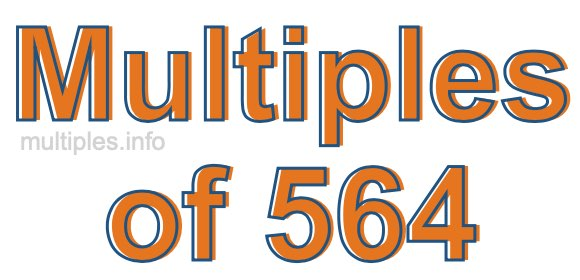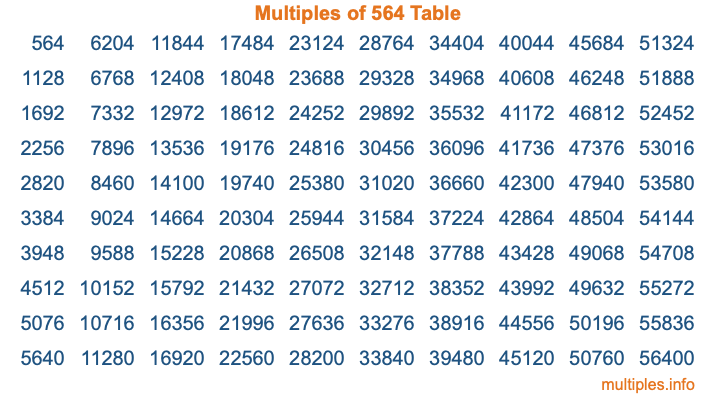Multiples of 564Welcome to the Multiples of 564 page. Here we will first teach you everything you will ever need to know about the multiples of 564, and then give you a study guide summary of everything we taught you to make sure you remember it all. Use this page to look up facts and learn information about the multiples of 564. This page will make you a multiples of five hundred sixty-four expert!

Definition of Multiples of 564
Multiples of 564 are all the numbers that when divided by 564 equal an integer. Each of the multiples of 564 are called a multiple. A multiple of 564 is created by multiplying 564 by an integer.

Therefore, to create a list of multiples of 564, you start with 1 multiplied by 564, then 2 multiplied by 564, then 3 multiplied by 564, and so on for as long as you want. Thus, the list of the first five multiples of 564 is 564, 1128, 1692, 2256, and 2820. To see a larger list of multiples of 564, see the printable image of Multiples of 564 further down on this page. We also have a category where you can choose any nth multiple of 564.

Multiples of 564 Checker
The Multiples of 564 Checker below checks to see if any number of your choice is a multiple of 564. In other words, it checks to see if there is any number (integer) that when multiplied by 564 will equal your number. To do that, we divide your number by 564. If the the quotient is an integer, then your number is a multiple of 564.

Is  a multiple of 564?

Least Common Multiple of 564 and ...
A Least Common Multiple (LCM) is the lowest multiple that two or more numbers have in common. This is also called the smallest common multiple or lowest common multiple and is useful to know when you are adding our subtracting fractions. Enter one or more numbers below (564 is already entered) to find the LCM.

Check out our LCM Calculator if you need more details about the Least Common Multiple or if you need the LCM for different numbers for adding and subtraction fractions.

nth Multiple of 564
As we stated above, 564 is the first multiple of 564, 1128 is the second multiple of 564, 1692 is the third multiple of 564, and so on. Enter a number below to find the nth multiple of 564.

th multiple of 564

Multiples of 564 vs Factors of 564
564 is a multiple of 564 and a factor of 564, but that is where the similarities end. All postive multiples of 564 are 564 or greater than 564. All positive factors of 564 are 564 or less than 564.

Below is the beginning list of multiples of 564 and the factors of 564 so you can compare:

Multiples of 564: 564, 1128, 1692, 2256, 2820, etc.

Factors of 564: 1, 2, 3, 4, 6, 12, 47, 94, 141, 188, 282, 564

As you can see, the multiples of 564 are all the numbers that you can divide by 564 to get a whole number. The factors of 564, on the other hand, are all the whole numbers that you can multiply by another whole number to get 564.

It's also interesting to note that if a number (x) is a factor of 564, then 564 will also be a multiple of that number (x).

Multiples of 564 vs Divisors of 564
The divisors of 564 are all the integers that 564 can be divided by evenly. Below is a list of the divisors of 564.

Divisors of 564: 1, 2, 3, 4, 6, 12, 47, 94, 141, 188, 282, 564

The interesting thing to note here is that if you take any multiple of 564 and divide it by a divisor of 564, you will see that the quotient is an integer.

Multiples of 564 Table
Below is an image of the first 100 multiples of 564 in a table. The table is in chronological order, column by column. The first column has the first ten multiples of 564, the second column has the next ten multiples of 564, and so on.The Multiples of 564 Table is also referred to as the 564 Times Table or Times Table of 564. You are welcome to print out our table for your studies.

Negative Multiples of 564
Although not often discussed or needed in math, it is worth mentioning that you can make a list of negative multiples of 564 by multiplying 564 by -1, then by -2, then by -3, and so on, to get the following list of negative multiples of 564:

-564, -1128, -1692, -2256, -2820, etc.

Multiples of 564 Summary
Below is a summary of important Multiples of 564 facts that we have discussed on this page. To retain the knowledge on this page, we recommend that you read through the summary and explain to yourself or a study partner why they hold true.

There are an infinite number of multiples of 564.

A multiple of 564 divided by 564 will equal a whole number.

564 divided by a factor of 564 equals a divisor of 564.

The nth multiple of 564 is n times 564.

The largest factor of 564 is equal to the first positive multiple of 564.

564 is a multiple of every factor of 564.

564 is a multiple of 564.

A multiple of 564 divided by a divisor of 564 equals an integer.

564 divided by a divisor of 564 equals a factor of 564.

Any integer times 564 will equal a multiple of 564.

Multiples of a Number
Here you can get the multiples of another number, all with the same attention to detail as we did for multiples of 564 on this page.

Multiples of
Multiples of 565
Did you find our page about multiples of five hundred sixty-four educational? Do you want more knowledge? Check out the multiples of the next number on our list!